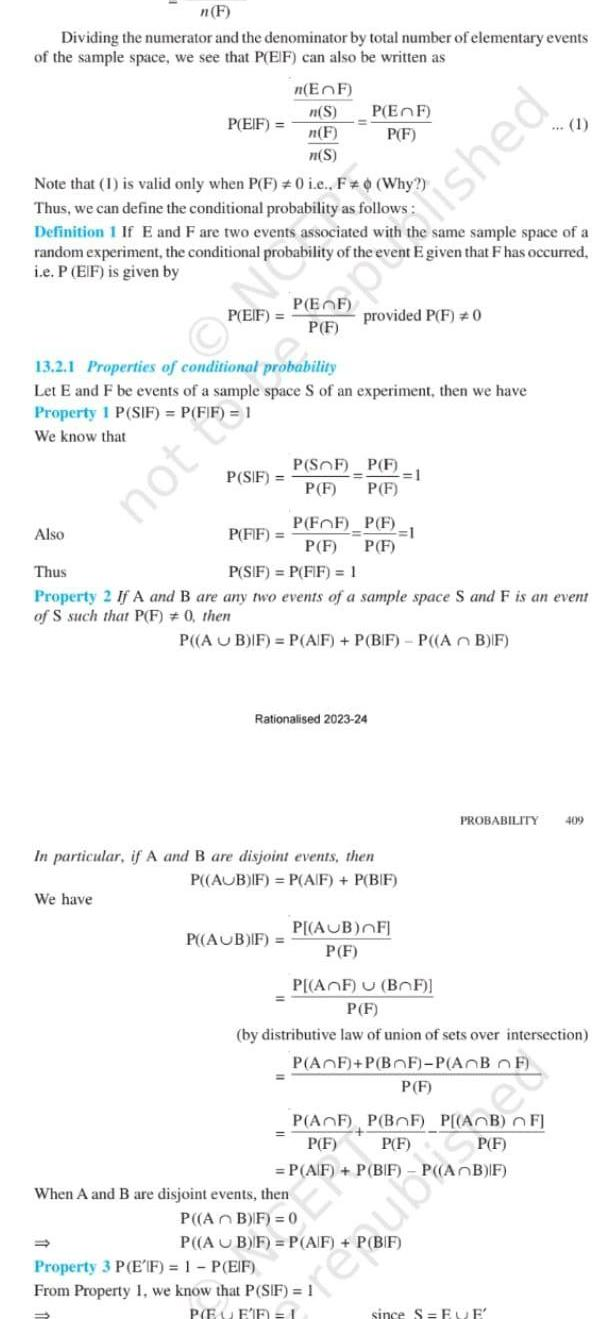Question:

# n F Dividing the numerator and the denominator by total

Last updated: 9/19/2023n F Dividing the numerator and the denominator by total number of elementary events of the sample space we see that P EIF can also be written as P EIF Also Note that 1 is valid only when P F 0 i e F Why Thus we can define the conditional probability as follows Definition 1 If E and F are two events associated with random experiment the conditional probability of the event E given that F has occurred i e P EIF is given by P EIF We have n EF n S n F n S P SIF 13 2 1 Properties of conditional probability Let E and F be events of a sample space S of an experiment then we have Property 1 P SIF P FIF 1 We know that P EF P F P FF P F P AUB IF P SOF P F P F P F provided P F 0 P FIF Thus P SIF P FIF 1 Property 2 If A and B are any two events of a sample space S and F is an event of S such that P F 0 then P AUB IF P A F P BIF P AB IF Rationalised 2023 24 P F 1 P F In particular if A and B are disjoint events then P AUB IF P A F P BIF P AUB FI P F same sample space of a Property 3 P EIF 1 P EIF From Property 1 we know that P SIF 1 P EEF 1 pride you P AF U BF P F by distributive law of union of sets over intersection P AF P BOF P AOB OF P F When A and B are disjoint events then P AB IF 0 P AB F P A F P BIF PROBABILITY 409 P AF P BF P AB FJ P F P F P F P A F P BIF P AB IF since SEVE